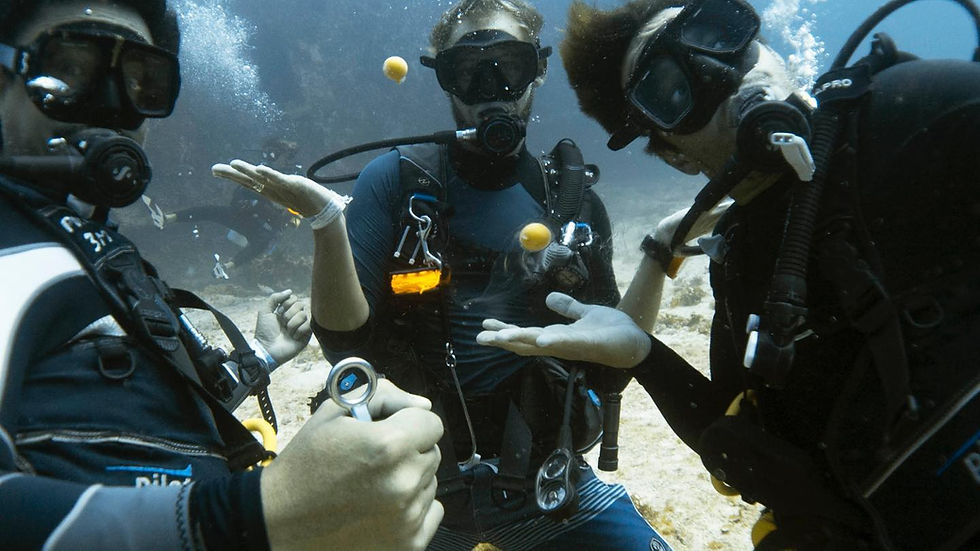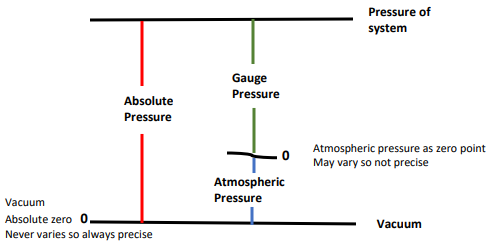top of page
Search
• Tracy Gunn

# Understanding Pressure in Physics IDC Dive Theory

Updated: Aug 20An egg held together by the pressure of the water

Pressure is important to us in diving for measuring how deep we are, how full our tanks are and what physiological effects our body will undergo, to mention a few examples. When we dive, we are subject to pressures greater than what we experience in our everyday life. These changes in pressure can cause various diving injuries, such as decompression sickness, lung over expansion and squeezes. Without an understanding of how pressure affects the body and how to deal with it, serious injury can occur.Pressure is defined as "continuous physical force exerted on or against an object by something in contact with it". We need to clearly understand this concept before we delve into the laws of Boyle, Charles, Henry and Dalton.

We are going to discuss here atmospheric pressure and water pressure.

The most basic pressure unit in diving is an atmosphere (atm) or bar. This is the pressure exerted by the atmosphere at sea level. While there is a slight difference between the two, it is minimal, and in diving, they are treated as equal.

Air pressure is known as atmospheric pressure (1)

Water pressure is known as hydrostatic pressure (2)

## Atmospheric pressure

Atmospheric pressure is the weight of the air above us, exerting pressure. Exactly how much pressure depends upon your altitude. The higher you are, the less air above you, hence the less dense the air and less atmospheric pressure. The lower you are, the more air above you, hence denser air and more atmospheric pressure.Atmospheric pressure is equal to the weight of one square-inch column of air extending all the way to the edge of the Earth´s atmosphere. The weight of this at sea level is 14.7 pounds, so the atmospheric pressure is 14.7 psi (pounds per square inch), or to talk in metric, a one square cm column will weigh 1.03 kg (1.03 kg/cm²).

For simplicities sake, this unit of measurement is called atmospheres or atm. 1 atmosphere is equal to 14.7 psi, 1.03kg/cm² or 760 mmHg

Calculating changes in atmospheric pressure is challenging (poor weather man) due to many varying conditions such as temperature and humidity, but luckily for us, water pressure changes constantly with depth as it does not compress like air. (6)

Because of the reduction in atmospheric pressure at altitude, special procedures apply for diving at altitude, and the use of special tables and theoretical depths are required.

How much does all this atmosphere weigh? Around 5.5 quadrillion tons, roughly one-millionth of the Earth`s mass. A cubic meter at sea level weighs 1.3 kg, and all the oceans together only weigh 270 times more than the atmosphere.

As discussed above, sea level air weighs 14.7 psi (metric 1.03 kg/cm²), and this means that there are about 15 pounds (6.8 kg) pushing down on every square inch (cm²) of you. In other words, that’s about the weight of a car pressing down on you all the time!

Luckily, we are not crushed because that weight is distributed over the entire planet and the surface of our body. Also, the amount of pressure exerted inward is in balance with the pressure exerted outward. This is because we breathe in the atmosphere too.

## What is meant by gauge, absolute and ambient pressure?The difference between gauge and absolute pressure is best explained like this. Absolute pressure uses absolute zero (perfect vacuum) as its zero point, while gauge pressure uses atmospheric pressure as its zero point. Because atmospheric pressure can vary, gauge pressure measurements are not precise, while absolute pressure is always definite. (3)

Absolute- If we desire to isolate a specific amount of pressure inside a process, regardless of what happens in the atmosphere, we should use a system of absolute pressure. (ata)

Gauge - If, however, we are concerned with maintaining a certain pressure relative to the current atmospheric pressure, then we should use a system of gauge pressure. (atm) (4)

Laws such as Boyle's and Dalton's require the use of absolute pressure to be able to calculate equations correctly.

## Gauge pressure

Gauge pressure ignores atmospheric pressure and is used when we need to measure pressure beyond the concerns of atmospheric pressure. Gauge uses the weight of the atmosphere (1 atm/bar or 14.7 psi) as the zero point. We need this to determine usable pressure, which is why it is the pressure reading used by most gauges.

For example, a gauge on an empty tank at the surface will read "0", and no air will flow out when you open the valve. However, there is still air in the cylinder (it is not a vacuum inside). Nothing flows out because the absolute pressure inside the tank is the same as the absolute pressure outside the tank (1atm/ bar or 14.7psi).

Your Submersible Pressure Gauge (SPG) is calibrated to read this way, ignoring the atmospheric pressure and reading "0" when the tank is "empty".

Your depth gauge is another example that calibrates to read "0" at the surface (sea level), ignoring atmospheric pressure and only measuring changes in water pressure.

An on-land example is tire pressure. This also subtracts the pressure of the atmosphere.

Gauge pressure is measured relative to the ambient atmospheric pressure and will change with variations to barometric pressure due to changes in weather. Because of this, it may not always be precise.

## Absolute pressure

"The definition of absolute pressure is the pressure of having no matter inside a space or a perfect vacuum. Measurements taken in absolute pressure use this absolute zero as their reference point. The best example of an absolute referenced pressure is the measurement of barometric pressure" (5)

Absolute pressure is the combination of atmospheric and water pressure, and both affect you while diving. The pressure change in water is much faster than in air, as water is around 830 times denser than air.

## Salt water vs fresh water

Because salt water has a different density than freshwater, pressure changes will occur faster, although pressure increases at a constant rate in both.

Surface = 1 atmosphere of pressure

Saltwater = 1 bar of pressure every 10 mt/33 ft

Freshwater = 1 bar of pressure every 10.3 mt/34 ft

Great news. This is printed on the front cover of your final exams.

Don´t mix this up with Archimedes' Principle.

## Absolute pressure in salt water

A 10-meter square cm column/ 33-foot square inch column of water exerts the same pressure as 1 atmosphere. Therefore, absolute pressure increases 1 atm every 10 mt/ 33 ft.

Because we need to take into account the one atmosphere at the surface, at 10 mt/ 33 ft, the pressure is 2 atmospheres. At 20 mt/ 66 ft, it is 3 atm and so on.

A common error in exams is that students forget to allow for the 1 atm of pressure at the surface. Absolute pressure is a combination of pressure from both water and air.

## Absolute pressure in freshwater

The density of fresh water is less than salt water, so the rate of pressure change differs slightly. Fresh water weighs less because there are less dissolved salts (less dense) (7). You need a little more depth in fresh water to have the same water pressure as salt water.

Pressure changes by 1 atm for every 10.3 mt/ 34 ft

To convert to another pressure measure, multiply by:

Metric Imperial

10 msw (meters of seawater) 33 for fsw (feet of seawater)

10.3 mfw (meters of fresh water) 34 for ffw (feet of freshwater)

1.03 for kg/cm² (kg per cm squared) 14.7 for psi (pounds per square inch)

## Ambient pressure

Ambient pressure means "surrounding pressure" and may be expressed as absolute (ata) or gauge (atm) pressure.

The surrounding pressure.

• On land, it comes from the weight of the atmosphere. (atm)

• At depth, it comes from the weight of the water plus the weight of the atmosphere (absolute pressure). (ata)

## THE EQUATIONS

To determine the pressure at depth, we need to establish three variables.

Depth: Meters or feet (up to you, but meters are easier)

Water: Saltwater of fresh water

Type of pressure requested: Ambient, absolute or gauge

Example 1

What is the Gauge pressure at 28 mt/92 ft of Fresh Water?

Metric: 28 m ÷ 10.3 m/atm = 2.7 atm gauge

Imperial: 92 ft ÷ 34 ft/atm = 2.7 atm gauge

What is the Absolute pressure at 28 mt/92 ft Fresh Water?

Metric: 28 m ÷ 10.3 m/atm + 1 atmosphere = 3.7 ata

Imperial: 92 ft ÷ 34 ft/atm + 1 atmosphere = 3.7 ata

What is the Gauge pressure at 28 mt/92 ft Salt Water?

Metric: 28 m ÷ 10 m/atm = 2.8 atm gauge

Imperial: 92 ft ÷ 33 ft/atm = 2.8 atm gauge

What is the Absolute pressure at 28 mt of Salt Water?

Metric: 28 m ÷ 10 m/atm + 1 atmosphere = 3.8 ata

Imperial: 92 ft ÷ 34 ft/atm + 1 atmosphere = 3.8 ata

Example 2

The pressure at 18mt/60 ft in salt water equals the same pressure at what depth in freshwater?

(depth = 0 at the surface, so this is a gauge pressure problem)

Metric: 18 mt ÷ 10 m/atm = 1.8 atm gauge

1.8 atm × 10.3 m/atm = 18.5 m

Imperial: 60 ft ÷ 33 ft/atm = 1.8 atm gauge

1.8 atm × 34 ft/atm = 61.2 ft

Example 3

What are the gauge and absolute pressure at a depth of 22.5 m/ 74 ft in freshwater?

What is the pressure in kg/cm² / psi?

Metric: 22.5 m ÷ 10.3 m/atm = 2.18 atm gauge

2.18 atm gauge + 1 atmosphere = 3.18 ata

2.18 atm × 1.03 kg/cm² = 2.24 kg/cm² gauge

3.18 ata × 1.03 kg/cm² = 3.28 kg/cm² absolute

Imperial: 74 ft ÷ 34 ft/atm = 2.18 atm gauge

2.18 atm gauge + 1 atmosphere = 3.18 ata

2.18 atm × 14.7psi/atm = 32 psi gauge

3.18 ata × 14.7 psi/atm = 46.7 psia

Remember: Always check the type of pressure requested (gauge, ambient or absolute), the type of water (sea or fresh) and the depth before starting the equation.## And to all the examsWater, Heat, Light, Sound, and Gases ExamArchimedes' Exam part 1Archimedes' Exam Part 2Under Pressure ExamBoyle's Law Exam Part 1

Single-Level Depth ChangesBoyle's Law Exam Part 2

Multi-Level Depth ChangesCharles' Law ExamHenry's Law ExamDalton's Law Exam

(1) Wikipedia contributors. (2021c, April 7). Atmospheric pressure. Wikipedia. https://en.wikipedia.org/wiki/Atmospheric_pressure

(2) What is Hydrostatic Pressure -- Fluid Pressure and Depth. (1999). EDinformatics. https://www.edinformatics.com/math_science/hydrostatic_pressure.htm#:%7E:text=Hydrostatic%20pressure%20is%20the%20pressure,exerting%20downward%20force%20from%20above

(3) J.M.K.C. Donev et al. (2020). Energy Education - Absolute pressure [Online]. Available: https://energyeducation.ca/encyclopedia/Absolute_pressure. [Accessed: April 15, 2021].

(4) Absolute vs gauge: choosing the right pressure control. (2021, January 27). Alicat Scientific. https://www.alicat.com/knowledge-base/absolute-vs-gauge-choosing-the-right-pressure-control/

(5) Difference Between Gauge and Absolute Pressure Measurement. (2013, June 18). ESI. https://www.esi-tec.com/blog-pressure-sensors-transmitter-transducer/2013/06/difference-between-gauge-and-absolute-pressure-measurement#:%7E:text=The%20definition%20of%20absolute%20pressure,the%20measurement%20of%20barometric%20pressure

(6) Willis, W. (2017).  Air Pressure – the weight of the gases in the atmosphere pushing on the surface of the Earth. - ppt download. Slide Player. https://slideplayer.com/slide/8328698/

(7) Is salt water heavier than fresh water? - Quora. (2018). Quora. https://www.quora.com/Is-salt-water-heavier-than-fresh-water

(6) Divemaster Course Instructor Guide (1999 edition). (2005). PADI.

(7) The Encyclopedia of Recreational Diving (3rd ed.). (2008). PADI.